View Course Path

# Code Converters – Binary to Excess 3, Binary to Gray and Gray to Binary

Next up in our digital electronics course, we will take a look at binary code converters. Let’s understand how code is encoded and converted between different forms. We will look at different types of code converters. By the end of this post, you will be able to design most of the important code converters, including a few important ones.

All the types of codes have some defining property in them. All of these codes are essentially used to help us encode decimal numbers in formats that can be understood by a digital system.

For a binary code, each bit has a value that depends on its position in the number.

Similarly, for gray code, its specialty lies in the fact that it requires each subsequent number to differ from its predecessor by only 1 bit. You can notice this when we derive the truth table below.

Whereas, the specialty of the Excess 3 code is that its value is +3 of that of its corresponding decimal place. 0 in decimal would be equivalent to 3 in excess 3 and so on.

We will see these specialties in detail in this post. We can inter-convert between these different types of codes using special combinational logic circuits known as code converters.

These different types of codes give us flexibility in designing transmission paths, memory storage, etc. So it’s cool to have them as options!

Contents

## How to design a 3-bit Binary to Gray Code Converter?

To convert a 3-bit binary to gray code, you need to follow a few rules/formulae.

The MSB of the binary code is always equal to the MSB of the gray code. The following bits of the gray code can be obtained by EXORing the corresponding binary bit and the preceding binary bit. If that’s confusing, just remember this figure below.Hence for 3-bit code converters,

G2 = B2

G1 = B1$\oplus$ B2

G0 = B0$\oplus$ B1

Based on the above equations, we can plot the following circuit diagram for a 3-bit binary to gray code converter using EX-OR logic gates.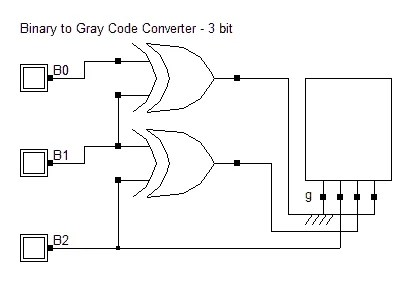### Truth Table for a 3-bit binary to gray code converter

 B2 B1 B0 G2 G1 G0 0 0 0 0 0 0 0 0 1 0 0 1 0 1 0 0 1 1 0 1 1 0 1 0 1 0 0 1 1 0 1 0 1 1 1 1 1 1 0 1 0 1 1 1 1 1 0 0

Note: Notice how each subsequent gray code number differs with its predecessor by only one bit.

## How to design a 4-bit Binary to Gray Code Converter?

The process is similar to the one we saw above. The MSBs are going to be equal. The subsequent gray code bit will be obtained by EXORing the corresponding binary bit with the preceding binary bit. Here’s a similar diagram to the one above to help you visualize the formula.Based on this

G3 = B3

G2 = B2$\oplus$ B3

G1 = B1$\oplus$ B2

G0 = B0$\oplus$ B1

The equations above indicate the presence of three EXOR gates. Therefore the simple combinational circuit for 4-bit binary to gray code converters is as shown below.### Truth Table for a 4-bit binary to gray code converter

 B3 B2 B1 B0 G3 G2 G1 G0 0 0 0 0 0 0 0 0 0 0 0 1 0 0 0 1 0 0 1 0 0 0 1 1 0 0 1 1 0 0 1 0 0 1 0 0 0 1 1 0 0 1 0 1 0 1 1 1 0 1 1 0 0 1 0 1 0 1 1 1 0 1 0 0 1 0 0 0 1 1 0 0 1 0 0 1 1 1 0 1 1 0 1 0 1 1 1 1 1 0 1 1 1 1 1 0 1 1 0 0 1 0 1 0 1 1 0 1 1 0 1 1 1 1 1 0 1 0 0 1 1 1 1 1 1 0 0 0

## How to design 3-bit Gray to Binary Code Converters?

To convert from gray to binary, a slightly different approach from the one we saw above is used. The MSBs are always equal. The next binary bit is obtained by EXORing the corresponding gray code bit with the preceding binary bit. Let’s take a look at a visual representation to get a clearer picture of the formula.Based on this

B2 = G2

B1 = G1$\oplus$ B2

B0 = G0$\oplus$ B1### Truth Table for a 3-bit gray to binary code converter

 G2 G1 G0 B2 B1 B0 0 0 0 0 0 0 0 0 1 0 0 1 0 1 0 0 1 1 0 1 1 0 1 0 1 0 0 1 1 1 1 0 1 1 1 0 1 1 0 1 0 0 1 1 1 1 0 1

## How to design a 4-bit Gray to Binary Code Converter?

Similar to the method above, a 4 bit gray to binary converter’s truth table can be prepared.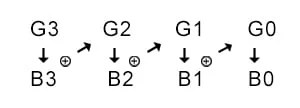B3 = G3

B2 = G2$\oplus$ B3

B1 = G1$\oplus$ B2

B0 = G0$\oplus$ B1

### Truth Table for a 4-bit gray to binary code converter

 G3 G2 G1 G0 B3 B2 B1 B0 0 0 0 0 0 0 0 0 0 0 0 1 0 0 0 1 0 0 1 0 0 0 1 1 0 0 1 1 0 0 1 0 0 1 0 0 0 1 1 1 0 1 0 1 0 1 1 0 0 1 1 0 0 1 0 0 0 1 1 1 0 1 0 1 1 0 0 0 1 1 1 1 1 0 0 1 1 1 1 0 1 0 1 0 1 1 0 0 1 0 1 1 1 1 0 1 1 1 0 0 1 0 0 0 1 1 0 1 1 0 0 1 1 1 1 0 1 0 1 1 1 1 1 1 1 0 1 0

The circuit obtained by olving the K-maps for the above truth table is as below.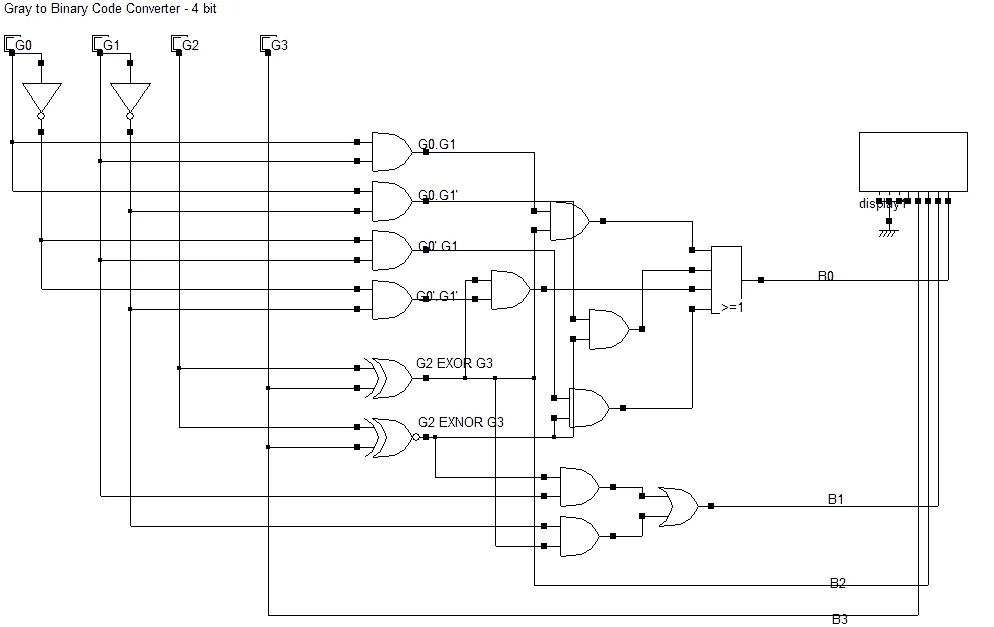## How to design a 3-bit Binary to Excess-3 Code Converter?

An excess 3 code, as can be predicted from its name, is an excess of three of the binary number. Simply add three to a binary number and represent that in binary form, that will be your excess 3/xs3 code. So 0000 in binary will be equivalent to (0+3=3) 0011 in excess 3.

Yes, the number is written in binary format, and that can be a source of confusion. Think of it this way. You have a normal number system. However, your friend wants to be unique and says that for him, a six will be equal to your three. The representation is the same. However, the values differ by three.

 B2 B1 B0 E2 E1 E0 0 0 0 0 1 1 0 0 1 1 0 0 0 1 0 1 0 1 0 1 1 1 1 0 1 0 0 1 1 1 1 0 1 x x x 1 1 0 x x x 1 1 1 x x x

Let’s obtain the equations for the code converter circuit using K-maps.E2 = B0 + B1 + B1’B2  OR  E2 = B0 + B1 + B2 (if you take cells 4,5,6,7) (both are correct)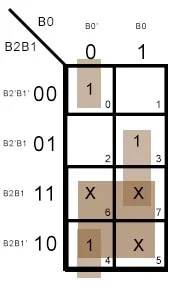E1 = B2 +$\overline { B0\oplus B1 }$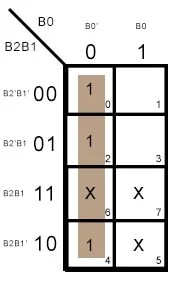E0 = B0′

Hence, from the equations above we can design the following combinational logic circuit for 3-bit binary to excess 3 code converter circuit.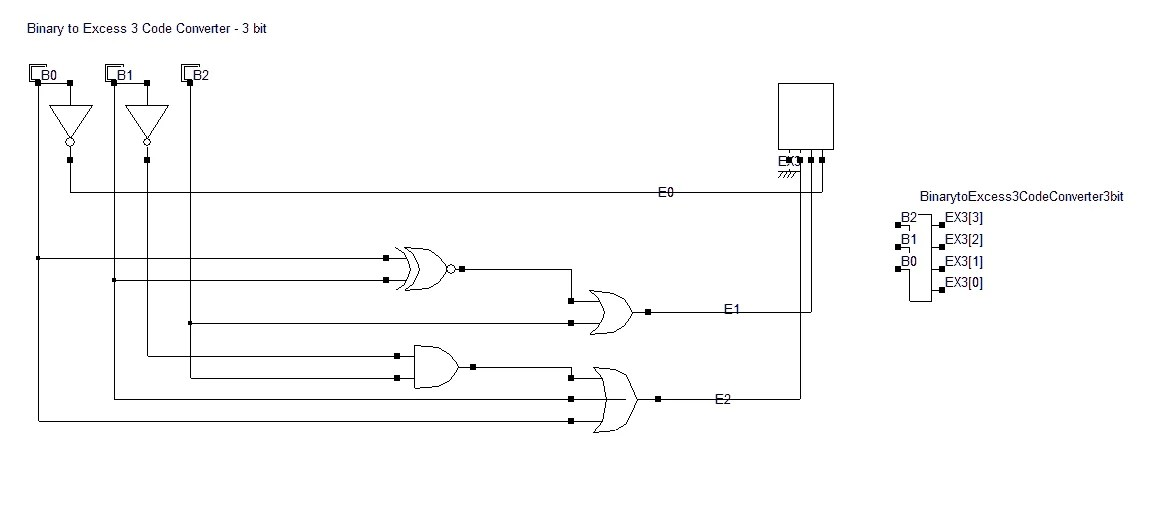## How to design a 4-bit Binary to Excess-3 Code Converter?

Following our footsteps from the designing of 3-bit binary to excess 3 code converters, we will first draft a truth table for the 4-bit version.

### Truth Table for a 4-bit binary to excess 3 code converter

 B3 B2 B1 B0 E3 E2 E1 E0 0 0 0 0 0 0 1 1 0 0 0 1 0 1 0 0 0 0 1 0 0 1 0 1 0 0 1 1 0 1 1 0 0 1 0 0 0 1 1 1 0 1 0 1 1 0 0 0 0 1 1 0 1 0 0 1 0 1 1 1 1 0 1 0 1 0 0 0 1 0 1 1 1 0 0 1 1 1 0 0 1 0 1 0 1 1 0 1 1 0 1 1 1 1 1 0 1 1 0 0 1 1 1 1 1 1 0 1 x x x x 1 1 1 0 x x x x 1 1 1 1 x x x x

Using Kmaps, we will solve for the output terminals. Remember that since we have don’t care conditions, there are more than one correct options for reducing the Kmap. You might end up with different equations than the ones in this post.

You should always try to optimize for the equation that requires you to employ the least number of logic gates.

This is because when you etch a logic circuit into an IC chip, the real estate of the chip is an expensive resource. And the least number of logic gates that you use, the higher will be the judicious use of space on the IC.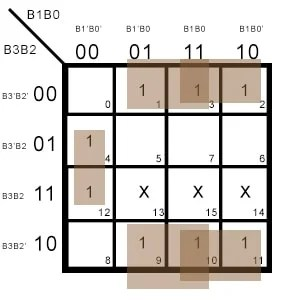E2 = B2′(B0 + B1) + B0’B1’B2E1 =$\overline { B0\oplus B1 }$

From the truth table

E0 = B0′

Using the above equations. we can draw the following logic circuit diagram for 4-bit binary to excess 3 code converters.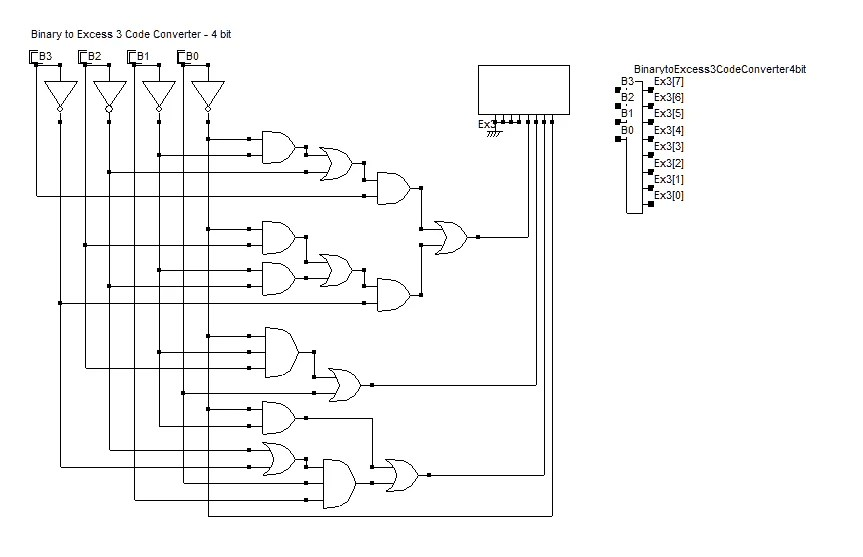Up next in our free digital electronics and digital logic design course, we are going to learn about the adders and the subtractors.

This site uses Akismet to reduce spam. Learn how your comment data is processed.